####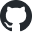https://github.com/xieguigang/voyager-1## 旅行者一号金唱片## 图像信号处理算法原理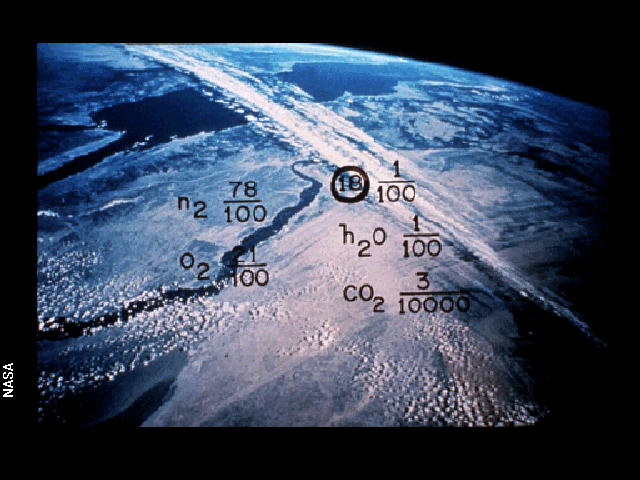Public Class ImageChunk

Public Property channel As ChannelPositions
Public Property start As Integer
Public Property length As Integer
''' <summary>
''' it is always [364,540] pixels?
''' </summary>
''' <returns></returns>
Public Property size As Size

''' <summary>
''' get wave data on left channel or right channel
''' </summary>
''' <param name="samples"></param>
''' <returns></returns>
Public Function GetSampleData(samples As IEnumerable(Of Sample)) As Single()
If channel = ChannelPositions.None OrElse channel = ChannelPositions.Left Then
Return samples.Select(Function(d) d.left).ToArray
Else
Return samples.Select(Function(d) d.right).ToArray
End If
End Function
End Class

Dim samples As Sample() = wav.data _
.ToArray
Dim data As Single() = chunk.GetSampleData(samples).PreProcessing

Dim align As Integer
Dim index As Integer = 0
Dim ncols As Integer = Math.Floor(data.Length / args.windowSize)
Dim buffer As Single()
Dim start, ends As Integer
Dim startOffset As Integer = args.windowSize * offsetLeft
Dim endOffset As Integer = (args.windowSize - args.windowSize * offsetRight)

For j As Integer = 0 To ncols - 1
buffer = New Single(args.windowSize - 1) {}

Call Array.ConstrainedCopy(data, index, buffer, Scan0, args.windowSize)

start = Which.Max(buffer.Take(startOffset))
ends = endOffset + Which.Min(buffer.Skip(endOffset))

' trim buffer
buffer = buffer.Skip(start).Take(ends - start).ToArray
align = Math.Floor((ends - start) / khzRate)
index += ends
aligns += align

Yield buffer.pixels(align, khzRate)
Next

<Extension>
Private Function pixels(data As Single(), align As Integer, khzRate As Integer) As Single()
Dim index As i32 = Scan0
Dim sum As Single() = New Single(khzRate - 1) {}

For j As Integer = 0 To sum.Length - 1
For i As Integer = 0 To align - 1
sum(j) += data(++index)
Next
Next

Return sum
End Function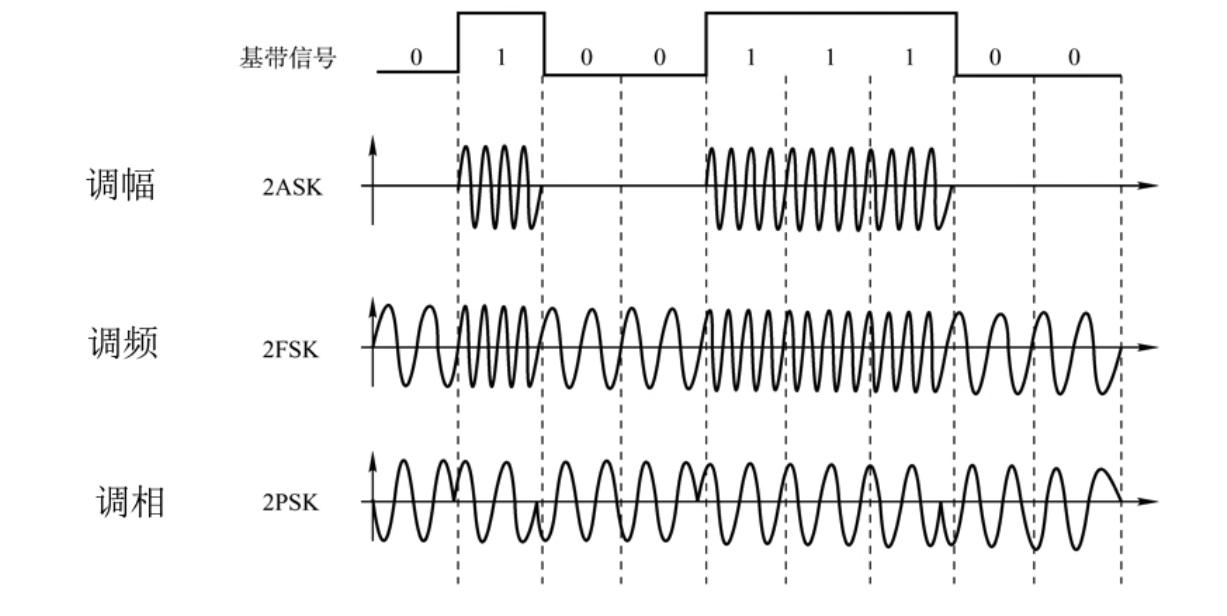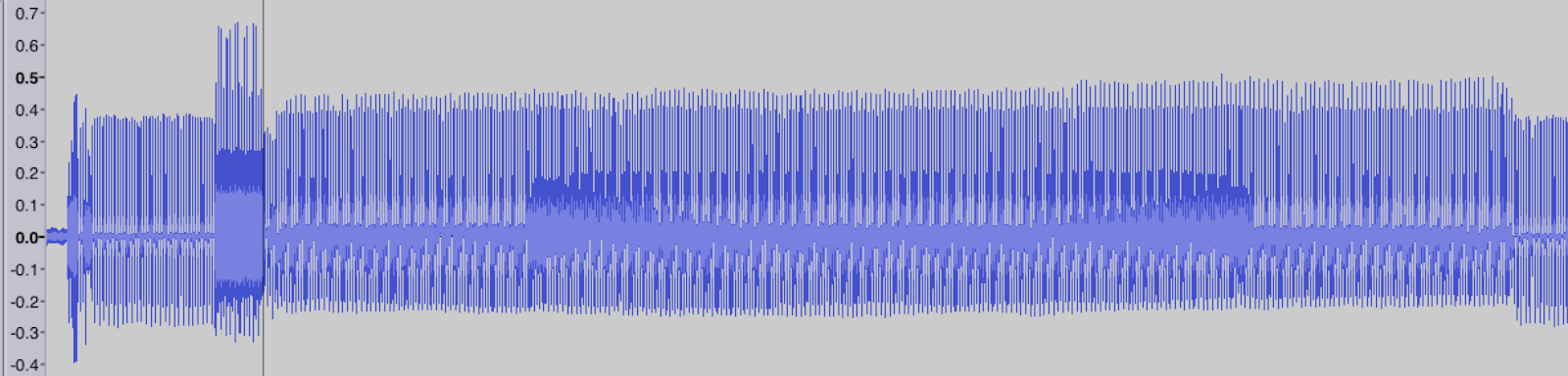Dim x As Integer = 0
Dim y As i32 = Scan0
Dim c As Color
Dim alignIndex As i32 = Scan0
Dim globalMax As Single = Aggregate col As Single()
In scans
Let colMax As Single = col.Max
Into Max(colMax)
Dim globalMin As Single = Aggregate col As Single()
In scans
Let colMin As Single = col.Min
Into Min(colMin)
Dim globalRange As New DoubleRange(globalMin, globalMax)
Dim alphaRange As DoubleRange = {0, 255}
Dim grayAlpha As Integer

Using img As BitmapBuffer = BitmapBuffer.FromBitmap(New Bitmap(width, khzRate, PixelFormat.Format32bppArgb))
For Each columnScan As Single() In scans
For i As Integer = 0 To columnScan.Length - 1
grayAlpha = globalRange.ScaleMapping(columnScan(i), alphaRange)
grayAlpha = 255 - grayAlpha

c = Color.FromArgb(grayAlpha, 0, 0, 0)

If y > img.Height - 1 Then
y = 0
x += 1
End If

If x > img.Width - 1 Then
x = 0
End If

' the data is a column scan
Call img.SetPixel(x, ++y, c)
Next
Next

Return img.GetImage
End Using## 通过R#脚本解码金唱片

imports "goldenRecord" from "voyager";

• decode 函数就是用来接收一个wav文件对象，然后从指定的位置开始读取一段数据然后解码为像素数据
• as.bitmap 函数就是将像素数据解码为最终的图像数据
• chunk_size 是一个调试测试算法用的函数，大家可以不用关心这个函数

bitmap(file = "/path/to/output.png") {
wav
|> decode(
chunk       = chunkPos,
decode      = decoder,
offsetLeft  = 0.15,
offsetRight = 0.1
)
|> as.bitmap()
;
}

• 选取好声音文件之中的数据块的位置
• 进行wav声音文件的decode操作
• 将数据块中的声音数据解码渲染为图像数据

## 解码出第一个圆require(voyager1);

imports "goldenRecord" from "voyager";
imports "wav" from "signalKit";

const goldenRecord as string = "J:\GoogleDrive\Voyager\384kHzStereo.wav";

# A demo R# script for image decode from the goden record wave data
# this very first circle image on the goden record is used for
# parameter calibration of the image decoder
using wav as read.wav(file = file(goldenRecord), lazy = TRUE) {
# view of the raw file data summary;
print(wav);

# parameters of the first circle image
# and wav decoder arguments
const first_circle = new image.chunk(
channel = "Left",
start   = 6000000,
length  = 1800000
);
const decoder = new decode(windowSize = 3400, offset = 384);

print(first_circle);
print("data size of this image chunk:");
print(wav :> chunk_size(chunk = first_circle));

# run decoder and save the
# result image file
bitmap(file = ${dirname(!script$dir)}/docs/circle.png) {
wav
|> decode(
chunk       = first_circle,
decode      = decoder,
offsetLeft  = 0.15,
offsetRight = 0.1
)
|> as.bitmap()
;
}
}• 接着我们就可以构建出解码图像所需要的image.chunk数据块信息，以及decode参数信息。
• 最后我们通过前面介绍的decode函数和as.bitmap函数就可以完整我们所设定的数据块的图像解码操作了。## 解码其他的图片

const data_chunks = list(
"Calibration_circle"        = 6000208,
"Solar_location_map"        = 8465560,
"Mathematical_definitions"  = 10686671,
"Physical_unit_definitions" = 13001000
);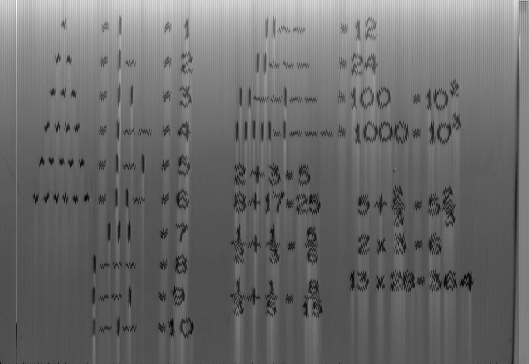Latest posts by 谢桂纲 (see all)

### Order by Date Name Attachments

1.2.3.4.5.